Previous in Forum: DC MOTOR Next in Forum: How Does a Zig-Zag Transformer Sense the Earth Fault?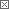Guru

Join Date: Sep 2007
Location: Defreestville, NY
Posts: 1072

#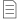A 4th Basic Circuit Element: The Memristor

05/01/2008 1:10 PM

In what could be the most significant invention since the transistor,scientists at HP labs have created a new circuit element, the memristor or a resistor with memory.

It won't be long until we have computers that boot up instantaneously.

http://www.nature.com/news/2008/080430/full/news.2008.789.html

What other applications can you suggest for this new device?

__________________
Charlie don't surf.
Interested in this topic? By joining CR4 you can "subscribe" to

### Comments rated to be Good Answers: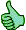These comments received enough positive ratings to make them "good answers".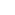#1

###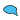Re: The 4th Circuit Element: The Memristor

05/01/2008 1:17 PM

Looks cool

Guru

Join Date: May 2007
Location: Annapolis, Maryland
Posts: 7266#2

###Re: A 4th Basic Circuit Element: The Memristor

05/01/2008 10:59 PM

I read the EE Times article and wondered to myself, why is the 'fourth' circuit element a non-linear device and the other three basic circuit elements (resistors, capacitors, and inductors) are linear devices?#7
In reply to #2

###Re: A 4th Basic Circuit Element: The Memristor

05/02/2008 9:29 PM

It seems to me that resistors, capacitors and inductors are only linear in their IDEAL states...Or maybe I am doing something wrong? Capacitors do not accept charge at a constant rate over time, inductors have a quick short-circuit spike when activated, followed by rather non-linear response when the core is saturated, and have you ever overloaded a resistor? Can that explosion be considered a linear response?

Off Topic (Score 5)
Guru

Join Date: May 2007
Location: Geelong, Australia
Posts: 1084#3

###Re: A 4th Basic Circuit Element: The Memristor

05/02/2008 12:02 AM

It looks like a potentially useful device, but I don't understand why it would be considered a new "basic" circuit element. You'd still model it using the 3 real basic elements and some terms to cover its history. Remember the tunnel diode.

__________________
If there's something you don't understand...Then a wizard did it. As heard on "The Simpsons".
Guru

Join Date: Nov 2006
Location: KnoxTN
Posts: 1485#4

###Re: A 4th Basic Circuit Element: The Memristor

05/02/2008 12:09 AM

"In what could be the most significant invention since the transistor,scientists at HP labs have created a new circuit element, the memristor or a resistor with memory."

Could this have any relation to Tom Bearden's negative resistor?

__________________
Do Nothing Simply When a Way Can be Found to Make it Complex and Wonderful
Anonymous Poster#5

###Re: A 4th Basic Circuit Element: The Memristor

05/02/2008 11:03 AM

Diodes are not considered a basic circuit element?4#6

###Re: A 4th Basic Circuit Element: The Memristor

05/02/2008 11:31 AM

Hi all. I too was confused about what made a memristor on the same level as a resistor, inductor, and capacitor (basic circuit elements). I found the following explanation on wikipedia:

The four fundamental circuit variables are current, I; voltage, V, charge, Q; and magnetic flux, Φm. Only 5 elements are required to represent any component or network by manipulating these four variables:

• Two sources:
• Current source, measured in amperes - produces a current in a conductor. Affects charge according to the relation dQ = − Idt.
• Voltage source, measured in volts - produces a potential difference between two points. Affects magnetic flux according to the relation dΦm = Vdt.
• Three passive elements:
• Resistance R, measured in ohms - produces a voltage proportional to the current flowing through it. Relates voltage and current according to the relation dV = RdI.
• Capacitance C, measured in farads - produces a current proportional to the rate of change of voltage across it. Relates charge and voltage according to the relation dQ = CdV.
• Inductance L, measured in henries - produces a voltage proportional to the rate of change of current through it. Relates flux and current according to the relation dΦm = LdI.

A fourth component, the memristor, has been proposed but is not generally accepted as one of the basic circuit elements. It was reported on April 30, 2008, that a working memristor has been developed by HP. It would have a memristance M, and would relate magnetic flux and charge by m = MdQ, so that every pair of variables would be related.

So if I'm reading this correctly, its considered a basic circuit element by some because it relates charge and magnetic flux, two of the fundamental circuit variables.

Given (V,I,Q,Φ), the possible combinations are:

V,I - Resistor
V,Q - Capacitor
V,Φ - Memristor
I,Q - Current Source
I,Φ - Inductor
Q,Φ - Voltage Source

Anyway, that's how I'm understanding it.

Good Answer (Score 4)
Member

Join Date: Nov 2008
Posts: 6#8
In reply to #6

###Unlike other circuit elements, Memristance is a function of charge

07/22/2009 11:37 AM

Given (V,I,Q,Φ), the possible combinations are:

V,I - Resistor
V,Q - Capacitor
V,Φ - Memristor
I,Q - Current Source
I,Φ - Inductor
Q,Φ - Voltage Source

I love the idea of memristance fitting into the relationships between voltage, charge, inductance, and flux, BUT I have a question about this:

• Q,V - Q=CV
• V, I --> V=RI
• I, Φ --> Φ=LI
• Φ, Q --> Φ=MQ

That's great. Let me analyze Φ=MQ. dΦ=M dQ. dΦ=v dt, so v dt = M dq. This means dq/dt =V/M. dq/dt = I, I=V/M. That's ohms law. The only difference between M and R is that M is a function of charge.

That's fine, but in all the other relationships, C, R, and L are constants. M, however, is a function of q. Does anyone know what the function is? Since M is a function, not a constant, isn't the relationship between flux and charge fundamentally different from the other relations.

Charles J Gervasi

Anonymous Poster#9
In reply to #6

###Re: A 4th Basic Circuit Element: The Memristor

09/12/2009 6:03 PM

I believe the relations are

V= dΦ/dt, voltage source

I = dq/dt, current source

V = k*I, resistor

V = k*q, capacitor

Φ = k*I, inductor

Φ = k*q, memristor

[I would say that V,q is a memristor and V,Φ is a voltage source]

Interested in this topic? By joining CR4 you can "subscribe" to